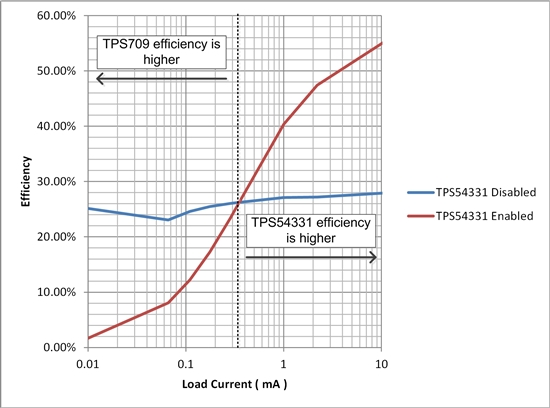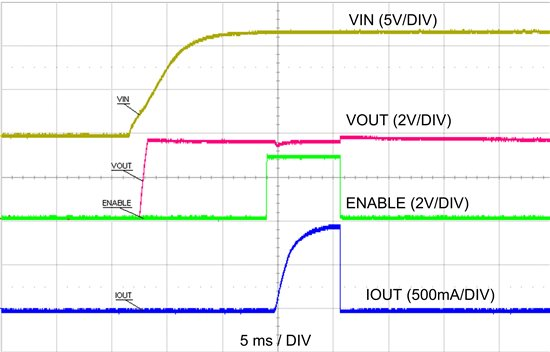# How-to Improve Buck Converter Light Load Efficiency with an LDO - Part 2

Part 1 of this series discussed the advantages of using an LDO in parallel with a DC/DC converter to optimize the no-load efficiency of the total power solution. In Part 2, I will discuss how this circuit was implemented with TI devices.

The circuit in figure 1 shows an example of efficiency improvement with an LDO in parallel with a DC/DC converter.Figure 1 – TPS709 in parallel with TPS54331

The LDO selected is the TPS709, which has a 30 V input range with only 1 µA nominal ground current.  The DC/DC converter is the TPS54331, selected for its input voltage range and high efficiency.  The TPS54331 already exhibits high efficiency at light loads in comparison to most comparable DC/DC converters.  However, at 110 µA (typical) non-switching quiescent current, the no-load current of the converter is nearly 100 times greater than the ground current of the TPS709 at no load (1.3 µA), even before switching losses and feedback resistor loads are factored in.

Figure 2 shows the efficiency measurement differences between the TPS709 and the TPS54331. The measurement configuration is VIN = 12 V, VOUT = 3.46 V when the TPS54331 is enabled, VOUT = 3.3 V when the TPS54331 is disabled.Figure 2 – Efficiency with the implemented circuit, TPS54331 Enabled and Disabled

As you can see, the efficiency of the TPS709 will remain roughly constant at 27% (VOUT/VIN) from 10 µA load current to 10 mA.  When the TPS54331 is enabled, it will start at a very low efficiency due to the no-load input current, but quickly get higher, peaking above 90% at higher load currents.  The TPS709 does not provide any load current when the TPS54331 is enabled, therefore, its ground current can be neglected.  From this, it can clearly be seen that it would be beneficial to disable the TPS54331 when the output load current is 350 µA or lower.

If the load circuit has a high-current slew rate when enabled, it may be necessary to delay the enable circuit to the load so that the DC/DC converter has sufficient time to be enabled before the load demands current from VOUT.  This can easily be done with an RC delay, however, care should be taken that the load does not remain on after the DC/DC is turned off.  A diode in parallel with the resistor can achieve this.  Figure 3 shows an example of this circuit.Figure 3 – TPS709 in parallel with TPS54331 with Load Enable Delay

Figure 4 shows the system response time scope shots of the system, along with the enable signal enabling the circuit into a 1A load.Figure 4 – Startup and Enable/Disable of Circuit in Figure 4

If you have any questions, please let me know in the comments below.